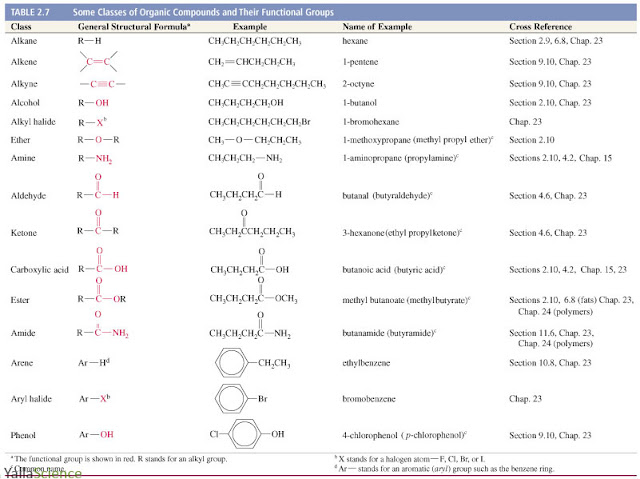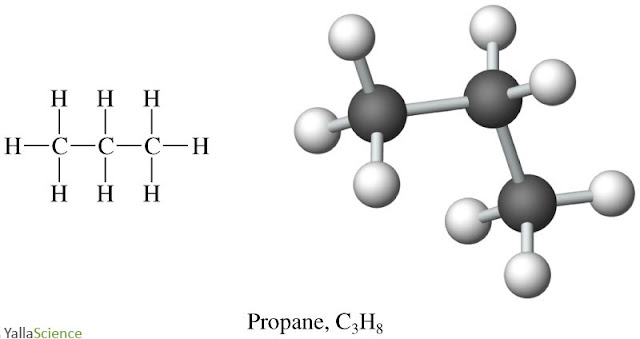# Chemistry Dictionary

## DonateName

Email *

Message *

## Weight percent w/w, w/v, v/v %

weight per weight (W/W), weight per volume (W/V), volume per volume (V/V) meanings and how to use it in chemistry

w/w , w/v, v/v %

These variations on percentage concentration are used in chemistry and biology when making up solutions and have the following meanings.

% w/w
weight per weight: used where the weight of each chemical is used and not the volume (e.g. If I dissolve 10 g of fat in 90 g ethanol so the total mass of the whole solution is 100 g, then I have made a 10% w/w solution of fat)

% w/v
weight per volume: used where a solid chemical is dissolved in a liquid (e.g. if I dissolve 10 g of table salt, sodium chloride, to make up a total volume of 100 mL of a solution then I have made a 10% w/v solution of sodium chloride)

% v/v
volume per volume: used where both chemicals are liquids (e.g. if I dilute 50 mL of acetic acid by adding it to 50 mL of water there is now 50 mL of acetic acid in a total volume of 100 mL, hence the acetic acid concentration is now 50% v/v

% volume per volume (v/v), % weight per volume (w/v) and % weight per weight (w/w)

Source:

Freefullpdf for Scientific Publications

## Some classes of organic compounds and their functional groups

Some classes of organic compounds and their functional groups

Fifteen of the classes of functional groups are shown, along with the general structural formula associated with that class. The key features are shown in red in the second columnSome classes of organic compounds and their functional groups

Tage: organic chemistry, structural formula, table

Source:
Freefullpdf for Scientific Publications

## Propane models

Propane models

Propane, used as a fuel, is shown in 3 different representations commonly used for molecules. Each format is used to convey different information about the structure and components of propane.Propane models

Tage: propane, ball and stick, models, fuel, molecular formula

Source:
Freefullpdf for Scientific Publications

## Ethane, minor component of natural gas

Ethane, a minor component of natural gas, is shown in representations. Each model conveys different information about the structure and components of ethane.Ethane, minor component of natural gas